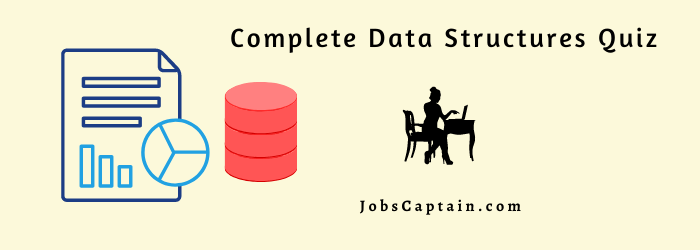# Data Structures MCQ Quiz 2 with Questions-AnswersPractices here Data Structures MCQ Quiz along with various topics like Array, linked list, tree, graphs, searching algorithms in data structure, and sorting algorithms in data structures.

## MCQ Quiz 2 – Data Structures Questions and Answers

Question 1: Collection of related data items is called ____________.

(A) files

(B) fields

(C) attributes

(D) records

(D) records

Question 2: Breadth First search is used in____________.

(A) binary tree

(B) stacks

(C) graphs

(D) both a and c

(C) graphs

Question 3: A variable whose size is determined at compile time and cannot be changed at run time is ______________.

(A) static variable

(B) dynamic variable

(C) not a variable

(D) data variable

(A) static variable

Question 4: Process of inserting an element in stack is called ____________.

(A) Create

(B) Push

(C) Evaluation

(D) Pop

(B) Push

Question 5: A technique for direct search is _______________.

(A) Binary Search

(B) Linear Search

(C) Tree Search

(D) Hashing

(D) Hashing

(A) first element

(B) middle element

(C) last element

(D) pivot element

(A) first element

Question 7: A _____________ list is a list where the last node contains null pointer.

Question 8: ___________are used to facilitate the processing of information in an array.

(A) Pointers

(B) Memory location

(C) Records

(D) Variables

(A) Pointers

Question 9: The comparison tree is also called as ______________.

(A) decision tree

(B) binary tree

(C) sequential tree

(D) b+ tree

(A) decision tree

Question 10: A linked list whose last node points back to the list node instead of containing the null pointer ____________.

(A) circular list

(A) circular list

Question 11: _________ is a header list where the last node contains the null pointer.

(D) Linear Array

Question 12: Which of the following case does not exist in complexity theory?

(A) Best case

(B) Worst case

(C) Average case

(D) Null case

(D) Null case

Question 13: The _________ for a linked list is a pointer variable that locates the beginning of the list.

(A) anchor

(B) base

(C) footer

Question 14: The time factor when determining the efficiency of algorithm is measured by ____________.

(A) counting microseconds

(B) counting the number of key operations

(C) counting the number of statements

(D) counting the kilobytes of the algorithm

(B) counting the number of key operations

Question 15: The space factor when determining the efficiency of algorithm is measured by___________.

(A) counting the maximum memory needed by the algorithm.

(B) counting the minimum memory needed by the algorithm.

(C) counting the average memory needed by the algorithm.

(D) counting the maximum disk space needed by the algorithm.

(A) counting the maximum memory needed by the algorithm.

Question 16: The Worst case occur in linear search algorithm when ___________.

(A) item is somewhere in the middle of the array.

(B) item is not in the array at all.

(C) item is the last element in the array.

(D) item is the last element in the array or is not there at all.

(D) item is the last element in the array or is not there at all.

Question 17: What is a linked list which always contains a special node called the header node, at the beginning of the list.

(B) Circular List

(D) None

Question 18: _______________is a header list where the last node points back to the header node.

Question 19: The advantage of a two-way list and a circular header list is combined into a ___________.

(B) two-way circular list

(D) None

Question 20: The pointer of the last node contains a special value called_____________.

(A) null pointer

(B) index pointer

(B) index pointer

Question 21: The OS of a computer may periodically collect all the deleted space onto the free storage list. This technique is called ______________.

(A) buffering

(B) garbage collection

(C) deal location

(D) buffer collection

Question 22: Important part of any compiler is the construction and maintenances of a dictionary, this types of the dictionary are called ___________.

(A) symbol table

(B) index table

(C) grammar table

(D) pointer table

(A) symbol table

Question 23: The data structure required to check whether an expression contains balanced parenthesis is _____________ .

(A) queue

(B) stack

(D) file

(B) stack

Question 24: What are the advantages of arrays?

(A) Easier to store elements of same data type

(B) Used to implement other data structures like stack and queue

(C) Convenient way to represent matrices as a 2D array

(D) All of the above

(D) All of the above

Question 25: The number of possible ordered trees with three nodes A,B,C is?

(A) 16

(B) 12

(C) 10

(D) 6

(B) 12

Question 26: The earliest use of__________ sorting was in conjunction with network analysis.

(A) topological

(B) bubble

(D) heap

(A) topological

Question 27: What is not the operation that can be performed on Queue?

(A) Traversal

(B) Insertion

(C) Deletion

(D) Retrieval

(A) Traversal

Question 28: A tree is a finite set of _____________.

(A) loops

(B) domains

(C) functions

(D) nodes

(D) nodes

Question 29: Stack can be represented by means of ____________.

(A) Tree

(B) Graph

(C) One-way List

(D) None of the above

(C) One-way List

Question 30: The hashing file space is divided into_______________.

(A) nodes and roots

(B) roots and slots

(C) buckets and slots

(D) slots and nodes